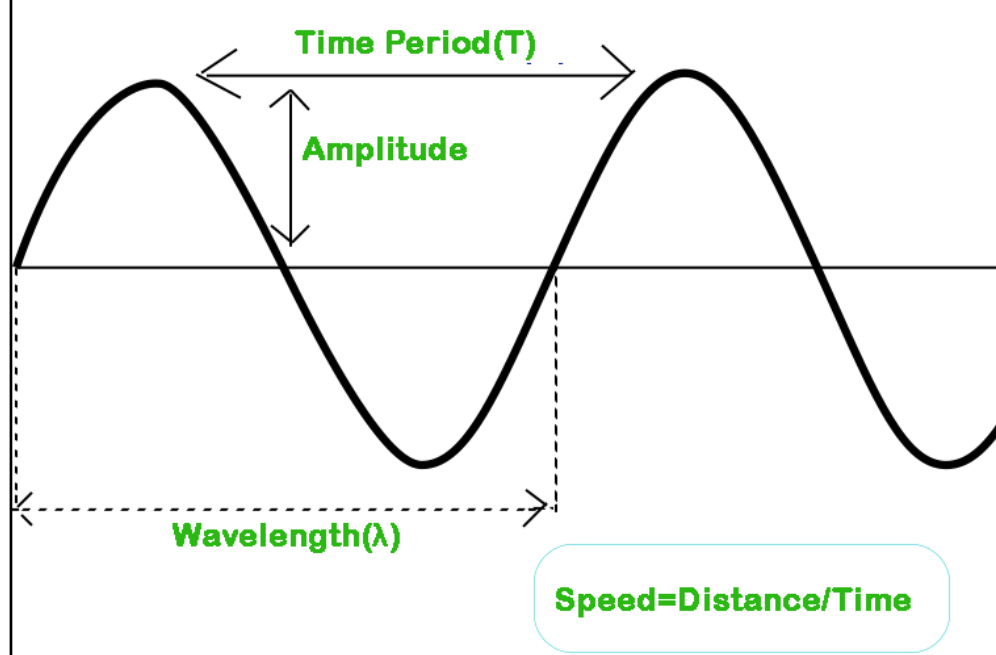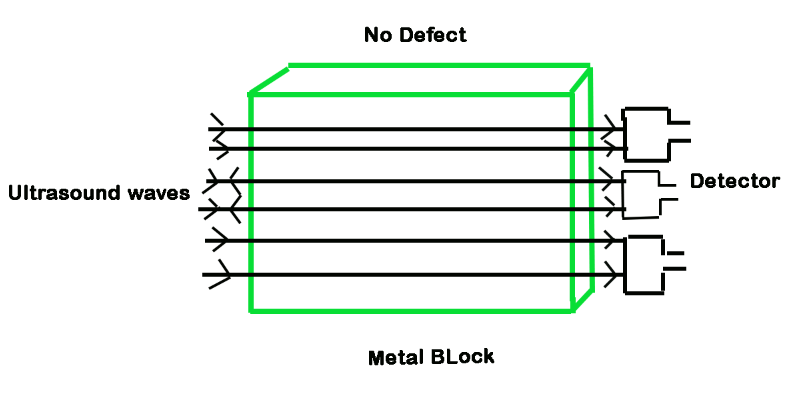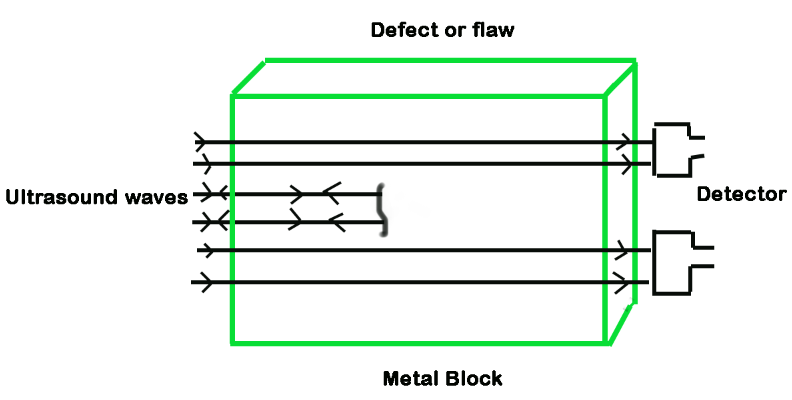Open in App
Not now

# Speed of Sound

• Last Updated : 26 May, 2021

Vibration or noise that passes through some medium is referred to as sound. It travels by moving energy from one molecule to the next and can be quickly heard as it enters a person’s ear. For example, when an object vibrates, it passes energy to the surrounding particles, causing them to vibrate as well. Since there are no particles to serve as a medium, sound cannot move through a vacuum. The sound may only flow through a medium such as water, air, or solid. We’ll talk about sound waves and the speed of the sound formula in this article. Let’s get started!

### What is a Sound Wave?

Sound is a wave that travels as longitudinal waves through air and liquid, but as both longitudinal and transverse waves through solid. The intensity at which a sound wave propagates is determined by the properties of the medium in which it travels. Its speed is independent of the wave’s characteristics or the force that produces it.

Its distribution in a medium may be used to investigate some of the medium’s properties. The speed of sound is defined as the distance traveled per unit of time by a sound wave propagating across an elastic medium. The speed of sound in a given medium is determined by its density and elasticity. According to mechanics, the greater the speed of sound, the greater the elasticity, and the lower the mass. Therefore, the speed of sound is maximum in solids and minimum in solids.

### Speed of Sound

Sound waves travel through a medium by contracting and expanding the medium’s parts as they travel. The speed of sound is defined as the distance traveled by any sound per unit of time. Let us now learn how to calculate the speed of sound in different mediums in the following section. The dynamic propagation of sound waves is known as the speed of sound.

This is determined by the properties of the medium in which the transmission occurs. The term “speed of sound” refers to the velocity of sound waves in an elastic medium. Hence, the speed of sound defines how quickly it can propagate in some medium. The speed depends on the medium. Speed of sound is measured as follows:Since, the general formula to calculate the speed is:

Speed = Distance / Time

Also, it is known that at any point of the wave travels a distance λ in time T.

Therefore, Speed is:

v = λ / T

= λf                                                                                                   (Since, the frequency f = 1 / T)

### Factors Affecting the Speed of Sound

The speed of sound is affected by two factors:

• Density of the medium: There is a need of medium for sound waves to travel, and the density of the medium is assumed to be one of the influences on which the speed of sound depends. When the medium is thick, the molecules in the medium are tightly packed, causing sound to propagate further. As a result, as the density of the medium increases, so does the speed of sound.
• Temperature of the medium: The temperature of the medium and the frequency of the sound waves are strictly proportional. As a result, as the temperature rises, so does the speed of sound.

### Speed of Sound in Air

The speed of sound is an important parameter in many fields of physics. The distance traveled per unit time by a sound wave propagating through a medium is referred to as the speed of sound.

At 20 °C, the speed of sound in air is 343.2 m/s, which corresponds to 1,236 km/h. At this rate sound will travel one mile in around five seconds. Sound travels 4 times faster in water (1,482 meters per second) and around 13 times faster through steel (4,512 meters per second).

### Speed of Sound in Different Media

The speed of sound depends on the properties of the medium through which it travels. The speed of sound decreases when we go from a solid to a gaseous state. In any medium, as we increase the temperature, the speed of sound increases.

Sound waves are mechanical waves that can only pass through matter. The medium is the matter in which the waves pass. The table below shows the speed of sound in various media. In general, sound waves fly the fastest through solids, then liquids, and finally gases. In gases, the speed of sound is equal to the square root of the absolute temperature (measured in Kelvin), but it is unaffected by the frequency of the sound wave, strain, or density of the medium. However, none of the gases we encounter in everyday life are ideal gases, and as a result, their properties vary slightly.

• Speed of Sound in Solid: Sound is nothing more than a vibration caused by particle collisions; one molecule colliding with the next, and so on. Solids are much denser than liquids or gases. This suggests that in solids, molecules are closer to each other than in liquids, and in liquids, molecules are closer to each other than in gases. Because of their near proximity due to mass, they will collide very easily. It takes less time for a stable molecule to collide with its neighboring molecule. Because of this advantage, the speed of sound in a solid is greater than that of a gas. The speed of sound in solid is 6000 m/s, while the speed of sound in steel is 5100 m/s. Another fascinating finding about sound speed is that sound moves 35 times faster in diamonds than in air.
• Speed of Sound in Liquid: A liquid’s density is higher than a gas’s density. As a result, the distances between molecules in liquids are greater than in solids but smaller than in gases. As a result, the speed of sound in liquids is intermediate between the speeds of sound in solids and gases.
• Speed of Sound in Gas: When sound approaches a liquid or solid, the speed of sound is independent of the density of the medium. Since gases expand to fill a given vacuum, their density is very uniform regardless of the type of gas. This is obviously not the case for solids and liquids.
• Speed of Sound in Water: The speed of sound in water exceeds that of air. Alternatively, sound moves quicker in water than in air. In water, the speed of sound is 1480 m/s. It’s also worth noting that the speed of purified water will range from 1450 to 1498 m/s, while the speed of seawater ranges from 1531 m/s while the temperature is between 20 °C and 25 °C.
• Speed of Sound in Vacuum: Since there are no particles in a vacuum, the speed of sound is zero metres per second. When there are ions for the propagation of these sound waves, they fly through a medium. Sound waves do not propagate in the vacuum since it is an empty space.

### Sample Problems

Problem 1: An echo is heard after 4 s. What is the distance of the reflecting surface from the source given that the speed of sound is 284 m/s?

Solution:

Given,

Speed of sound (v) = 284 m/s and

Echo returns in time (t) = 4 s

Therefore,

The distance traveled by sound:

d = v × t

= 284 m/s × 4 s

= 1136 m

Since, Sound has to travel a distance that is twice the distance of the reflecting surface and the source.

Hence, the distance of the reflecting surface from the source,

D = 1136 m / 2

= 568 m

Problem 2: A person clapped his hands near a cliff and heard the echo after 3 s. What is the distance of the cliff from the person if the speed of the sound is taken as 348 m/s?

Solution:

Given,

Speed of sound, v = 348 m/s and

Time taken for hearing the echo, t = 3 s.

Distance traveled by the sound,

d = v × t

= 348 m/s × 3 s

= 1044 m

In 2 s sound has to travel twice the distance between the cliff and the person.

Hence, the distance between the cliff and the person,

D = 1044 m / 2

= 522 m

Problem 3: Explain how defects in a metal block can be detected using ultrasound.

Solution:

Ultrasound waves have a high frequency. They can travel in mediums even with obstacles. Ultrasound waves can be transmitted through a metal block, if a defect is present then either its speed will change due to the defect or the wave will reflect back completely and will be detected by the detector. If no defect is found then the sound comes out at the expected velocity.Problem 4: A sound wave has a frequency of 4 kHz and wavelength 35 cm. How long will it take to travel 2.5 km?

Solution:

Given,

Frequency (ν) = 4 kHz = 4000 Hz

Wavelength (λ) = 35 cm = 0.35 m

We know that,

The speed of the wave = wavelength × frequency

v = λ ν

= 0.35 m × 4000 Hz

= 1400 m/s

The time taken by the wave to travel a distance of 2.5 km,

t = 2500 / 1400

=1.78 s

Thus, the sound will take 1.78 s to travel a distance of 2.5 km.

Problem 5: A body is vibrating 48000 times in one minute. If the velocity of sound in air is 360 m/s, find:

(a) Frequency of vibration in hertz,

(b) Wavelength of the wave produced.

Solution:

(a) Given,

Number of vibration in one minute = 48000

Number of vibrations in one sec =48000/60 = 800Hz

Therefore, Frequency (f)= 800 Hz

(b) Given,

Velocity of speed in air (v) = 360 m/s

Frequency (f) = 800 Hz

v = f λ

or λ = v / f

= 360 / 800

= 0.45 m

My Personal Notes arrow_drop_up